# 机器学习(分类，回归，聚类)评价指标

#### 机器学习(分类，回归，聚类)评价指标

• 混淆矩阵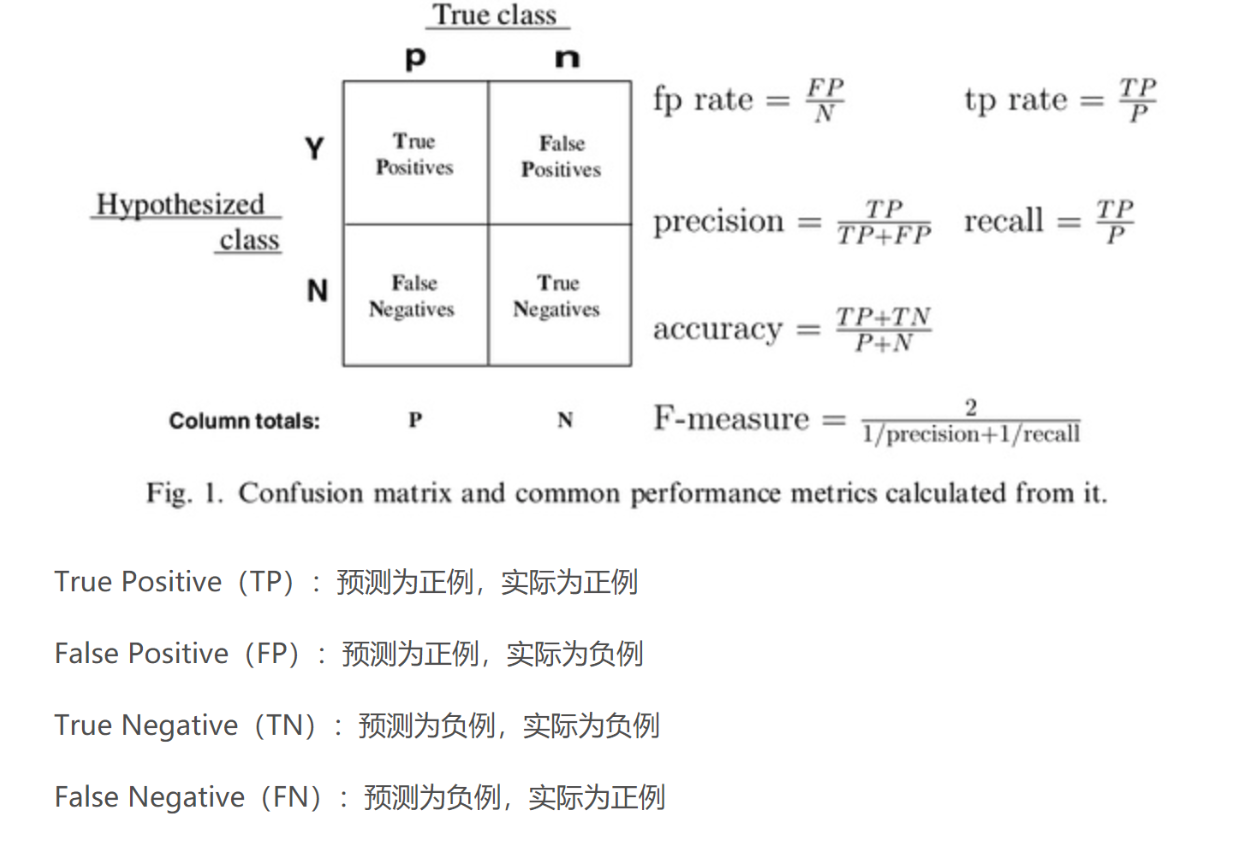• 精确率（accuracy）

正确预测的样本数占总预测样本的比值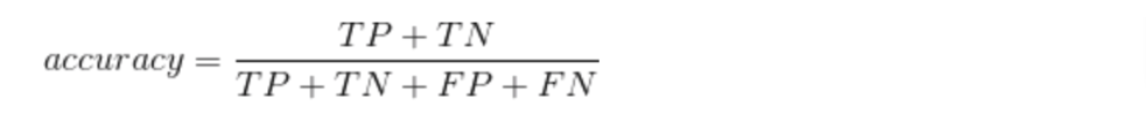• 召回率（recall）

也称为查全率，正确预测的正样本数占真实正样本数量比值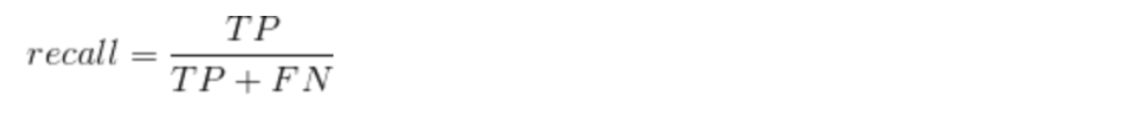• 准确率

正确预测的正样本数占所有预测为正样本的数量比值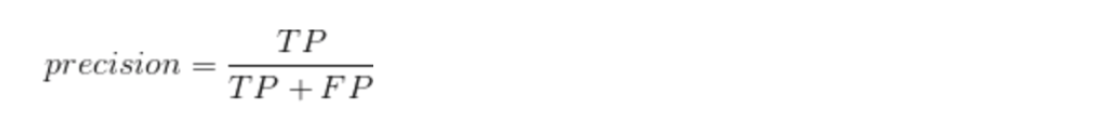• F值

正确率与召回率的平均调和

F值 =$\frac{precision*recall*2}{precision+recall}$

有时候会根据偏好为recall与precision设置不同权值：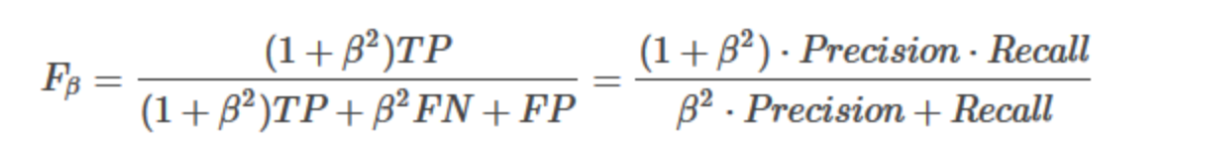• ROC-AUC

1. ROC（接收操作者特征曲线）

最早用于雷达信号检测领域，用于区分信号与噪声。现用来评价模型的预测能力，ROC曲线基于混淆矩阵得出的。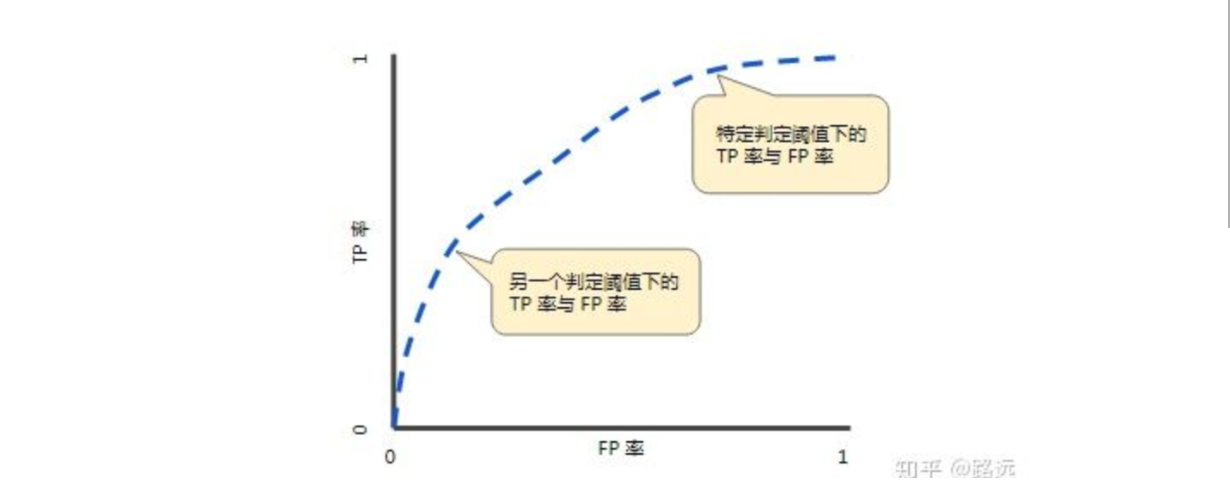两个指标：真阳率（TPR）为纵坐标与假阳率（FPR）为横坐标

TPR越高，同时FPR越低（即ROC曲线越陡），模型性能越好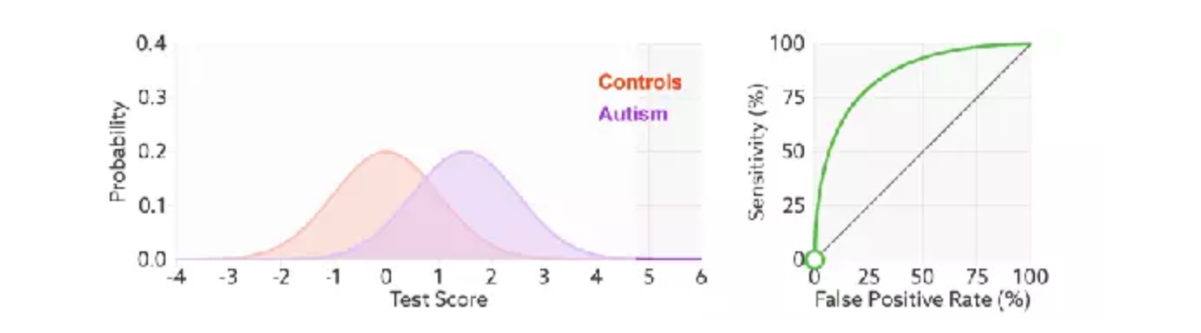2. AUC（曲线下的面积Area under curve）

ROC对角线实际含义：随机判断正负样本覆盖均为50%，表示随机效果。但实际上ROC越陡，效果越好；AUC一般是介于0.5~1之间。

0.50.7：效果不好，0.70.85：效果一般，0.850.95：效果较好，0.951:效果非常好

• PRC（precision-recall curve）

准确率与召回率之比，在正负样本分布得极不均匀(highly skewed datasets)的情况下，PRC比ROC能更有效地反应分类器的好坏。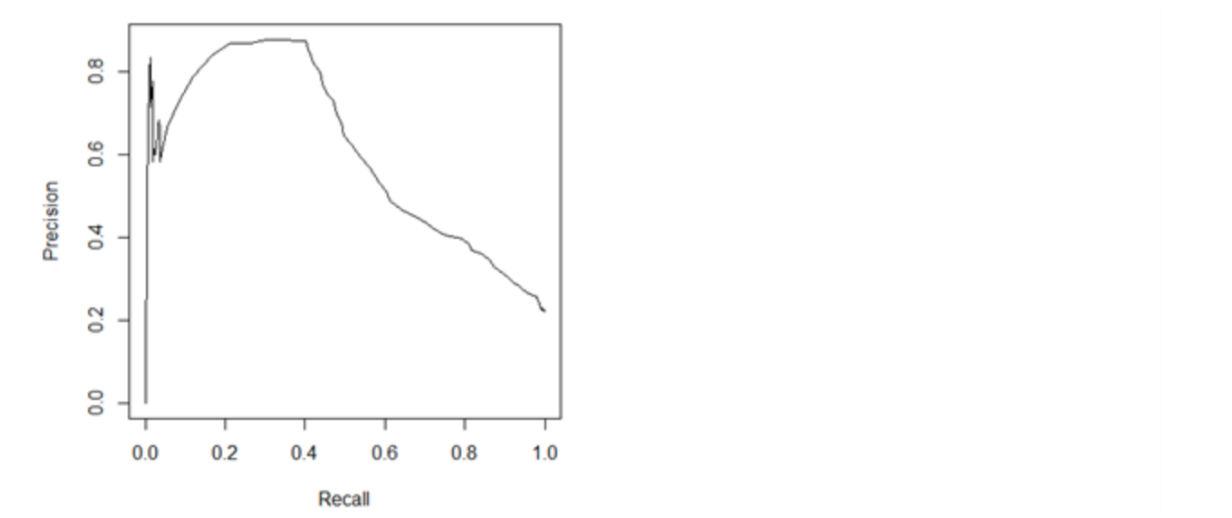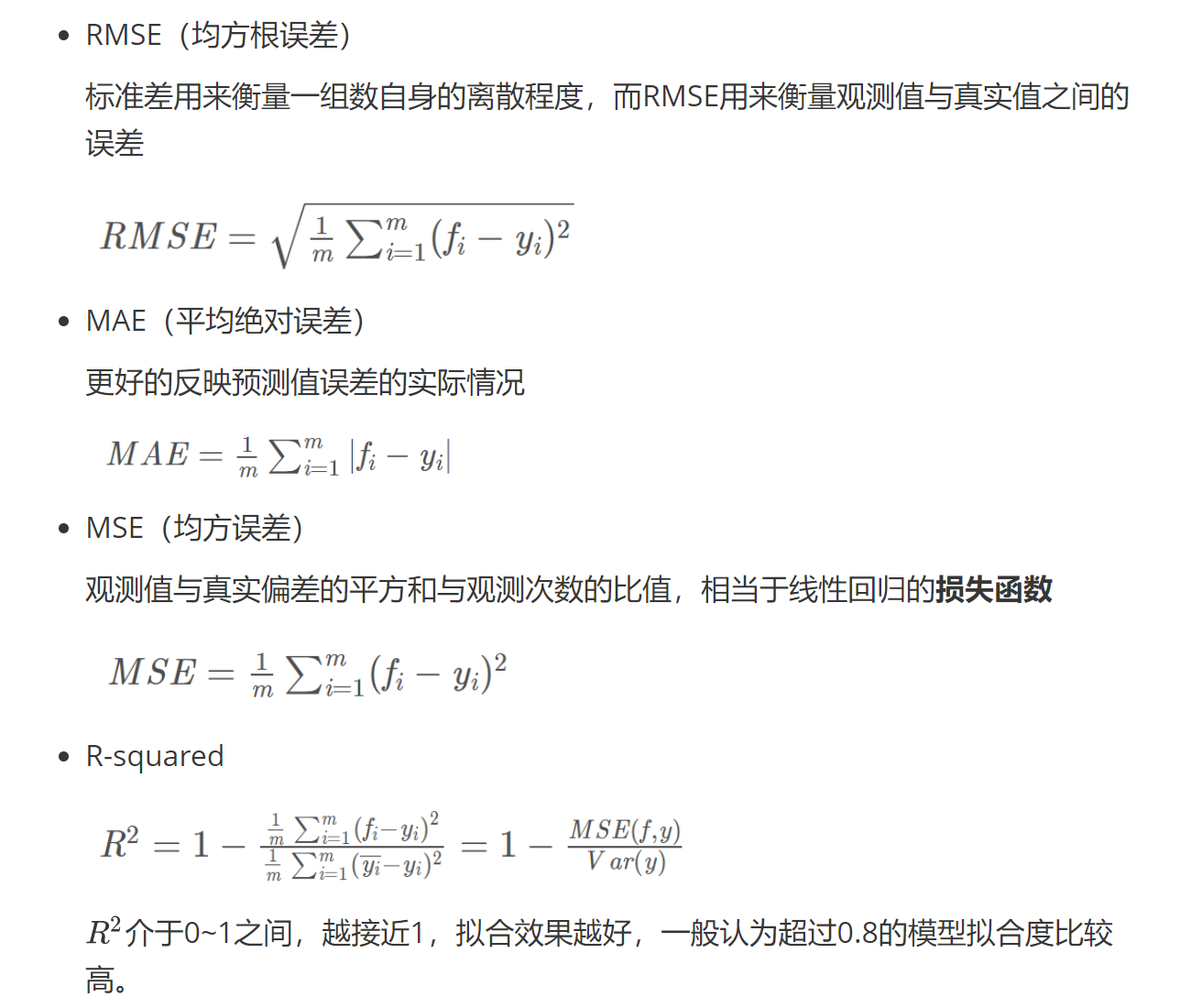1.如含标签

• 兰德指数

衡量两个分布的吻合程度，取值范围[-1,1],数值越接近1越好，聚类结果随机产生时，指标接近0.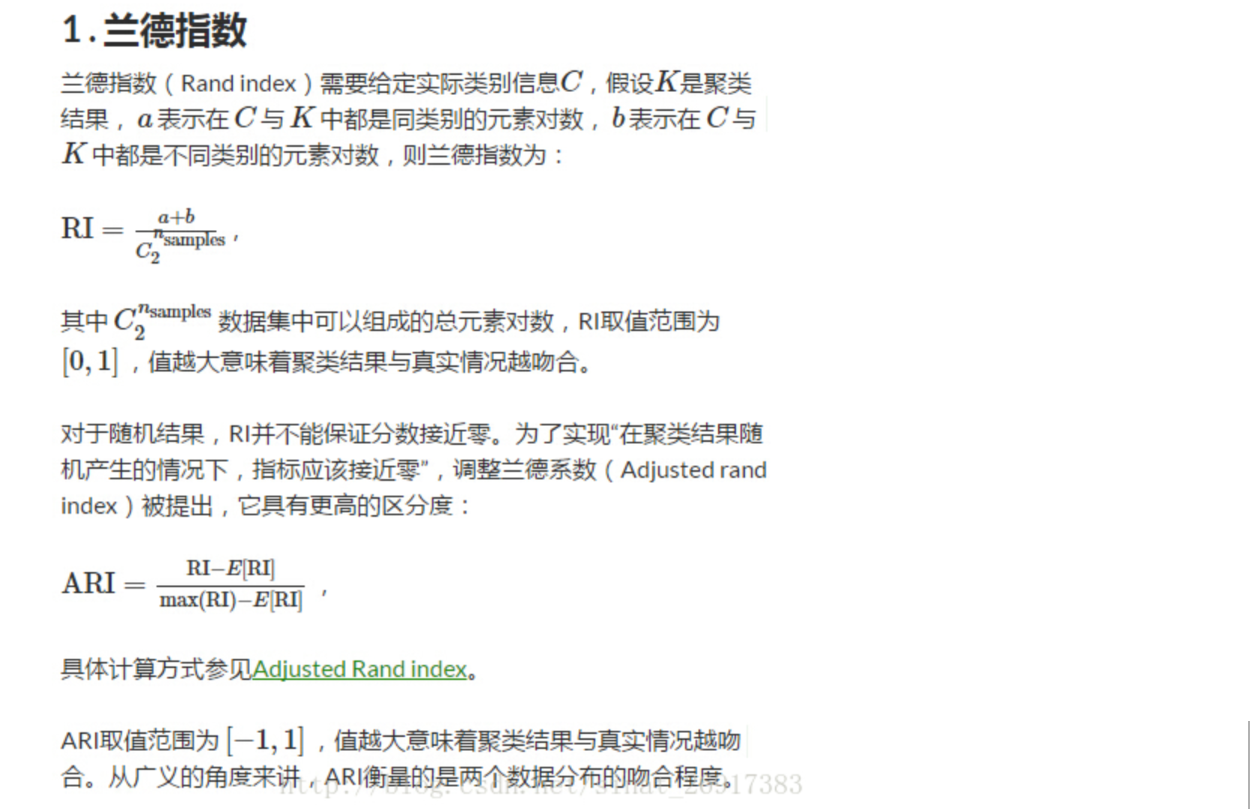• 互信息
衡量两个分布的吻合程度，取值范围[-1,1]，值越大聚类与真实效果越吻合。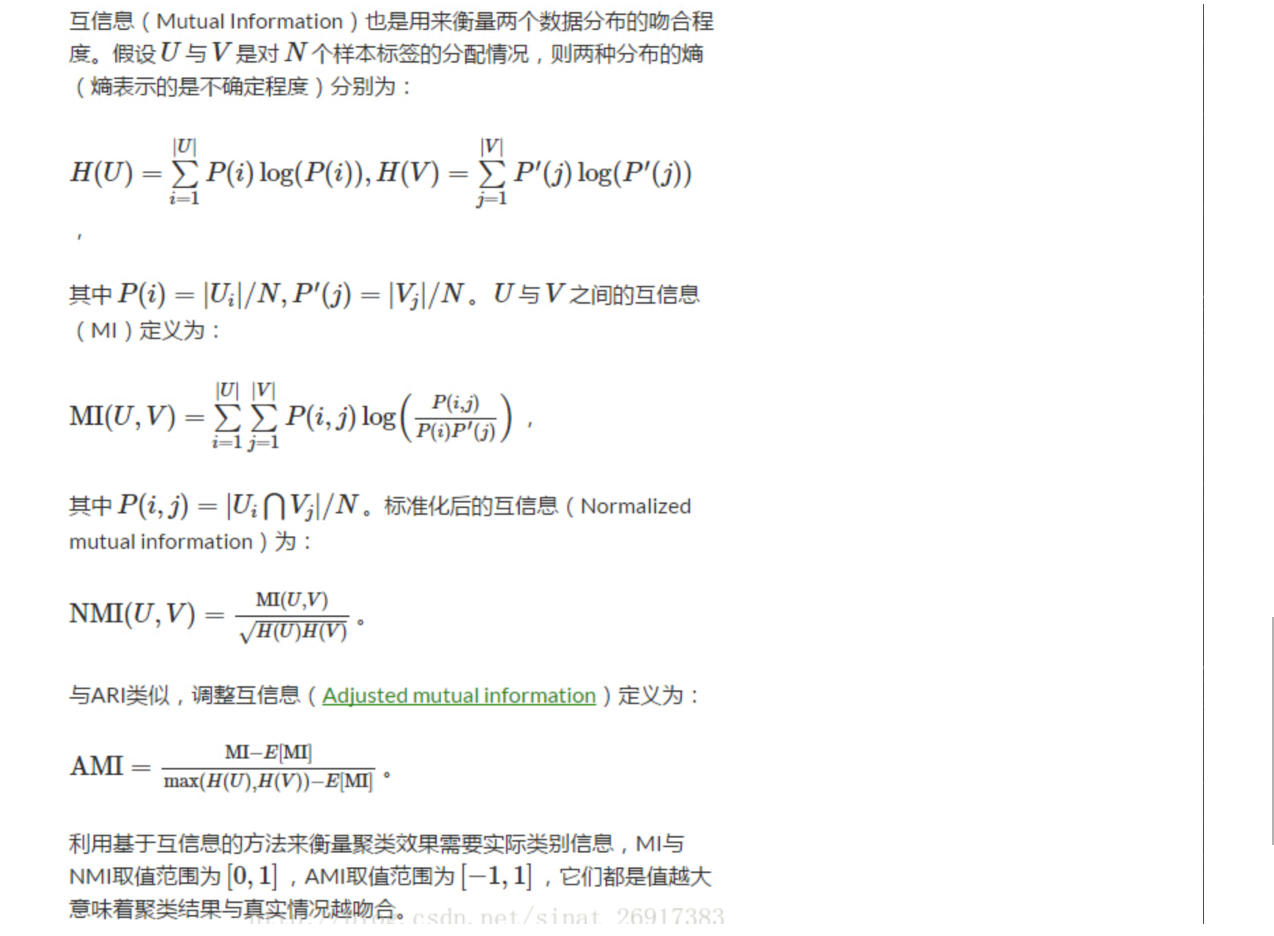• 轮廓系数

轮廓系数用来选择适合的聚类数目。根据折线图可以找到系数变化的幅度最大的点，发生畸变幅度最大的点就是最好的聚类数目。范围[-1,1].

对于聚类中的点$i$:

• 簇内不相似度$a(i)$ $i$向量到同簇内其他点不相似程度平均值，体现凝聚度

• 簇间不相似度$b(i)$$i$向量到其他簇平均不相似程度最小值，体现分离度

• 那么该对象的轮廓系数:• $S(i)$接近1，分类合理，接近-1.，分类错误，接近0，处于两个簇边界上。

2.不含标签

$WSS=\sum_{i=1}^{m}d(p_i,q^i)^2=\sum_{i=1}^{m}\sum_{j=1}^{n}(p_{ij}-q^i)^2 \\ BSS-\sum_{k=1}^{K}|Z_k|d(Q,q_k)^2=\sum_{k=1}^{K}\sum_{j=1}^{n}|Z_k|(Q_j-q_{kj})^2$

$q_{i}=(q_{1}^{i},q_{2}^{i} \dots q_{n}^{i})$,表示记录$i$所在聚类中心点的特征向量

K 聚类总数, $Z_k$k聚类中的记录数目

$Q=(Q_1,Q_2 \dots Q_n)$, 所有记录点特征向量

$q_k=(q_{k1},q_{k2}\dots q_{kn})$,表示第$k$哥聚类中心点特征向量

WSSBSS分别度量相同聚类内部记录之间的不相似度和不同聚类间记录的不相似度。显然，WSS越小，BSS越大，聚类结果越好。

©️2019 CSDN 皮肤主题: 大白 设计师: CSDN官方博客